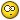1. Multiple Resistors instead of One

I was researching networks (N250TiBQ) for example, and saw two (R-1 and R-2) 82 ohm resistors, wired in parallel. I've seen the same practice (Multiple/Parallel Resistors) of the same value, in other networks. What are the advantages of this, compared to using one resistor?Reply With Quote

2. Double the power handling, typically. If 10-Watt resistors, the equivalent of one 20-Watt, 41-Ohm resistor.

Also, through averaging, a way of statistically narrowing the value tolerance range, though that's not usually an issue in crossovers....Reply With Quote

3. 0ne half the resistance with twice the power handling. You can also parallel up different values to get nonstandard values of resistance.

RobReply With Quote

4.Originally Posted by rek50
I was researching networks (N250TiBQ) for example, and saw two (R-1 and R-2) 82 ohm resistors, wired in parallel. I've seen the same practice (Multiple/Parallel Resistors) of the same value, in other networks. What are the advantages of this, compared to using one resistor?
Each resistor carries only half the current. If a resistor is rated for 10 watts, two would up the rating to 20 watts. This makes them run cooler reducing thermal change. Also redundancy of components may put you closer to the desired value as they tend to average out. Finally, some values are just not available and must be made up from others.Reply With Quote

5. Hi Robh3606

Yes. You can parallel resistors together to get nonstandard values of resistance. After doing a quick Google Search, I found the following site: http://www.1728.com/resistrs.htm

It is a little resistance calculator program, that I think you should find helpful.

There is one another advantage to using parallel resistors. Any inductance within each resistor is paralleled as well. This means that the inductance within the overall circuit is reduced.

So, it is possible to use several cheap wire-wound resistors in parallel and get results that would equal that of using the far more expensive "Audio Grade" non-inductive resistors.

Then again, you can improve on the quality of "Audio Grade" non-inductive resistors by using several of them in parallel.

Baron030Reply With Quote

6. Another good reason to use two resistors is that some values are not made and using two resistors in parallel can produce a value that is unique. it is not always an identical pair.

10 ohms and 22 ohms in parallel gives 6.8 ohms with high power handling ( for example) and if you place a variable resistor along side a fixed resistor you can get a narrow range of adjustment compared to the variable resistor alone.

One rule if thumb is the two resistors always produce a smaller value of resistance and more than 3 is seldom used because any desired value can be derived from three resistors.

R1 X R2
________ Gives value for two in parallel
R1 + R2

1 / 1/R1 + 1/R2 + 1/R3 Gives values for 3 resistors.Reply With Quote

7. Thanks all, for the input. Nice calc Baron030, I have it already, but it may be useful to others. Now all I have to find is a "Reverse" calc for resistors, if one exists. Plug in the desired value and see what parallel combinations would yeild said value, like 75 ohms for instance. The "Quest" continues...Reply With Quote

8. just don't expect to double the power handling if the values are mixed, especially
if they're not anywhere close in value to each other (the lower value resistor will
"take the heat" ... literally)... and if you can, leave a bit of air around them if power
dissipation is the goal (more effectively available surface area) or use thermal grease
and mount them to something thermally conductive to act as a heat sink.

Cheers,

-grumpyReply With Quote

9. You can solve for the missing value by substituting the desired value and reversing the calculations.Reply With Quote

10. Yes duaneage, the formula can be changed. I tried it out a couple of times to find a combination that would yeild 5.1 ohms. Maybe I'm slow, but the "Magic" combination didn't jump out at me. Give it a try and see how "Fast" it is5.1 = (X x Y) divided by (X + Y)
But then again, my Algebra "Brain Cells" were established in the 60's, followed by,well, I know I was there, but I forget....Reply With Quote